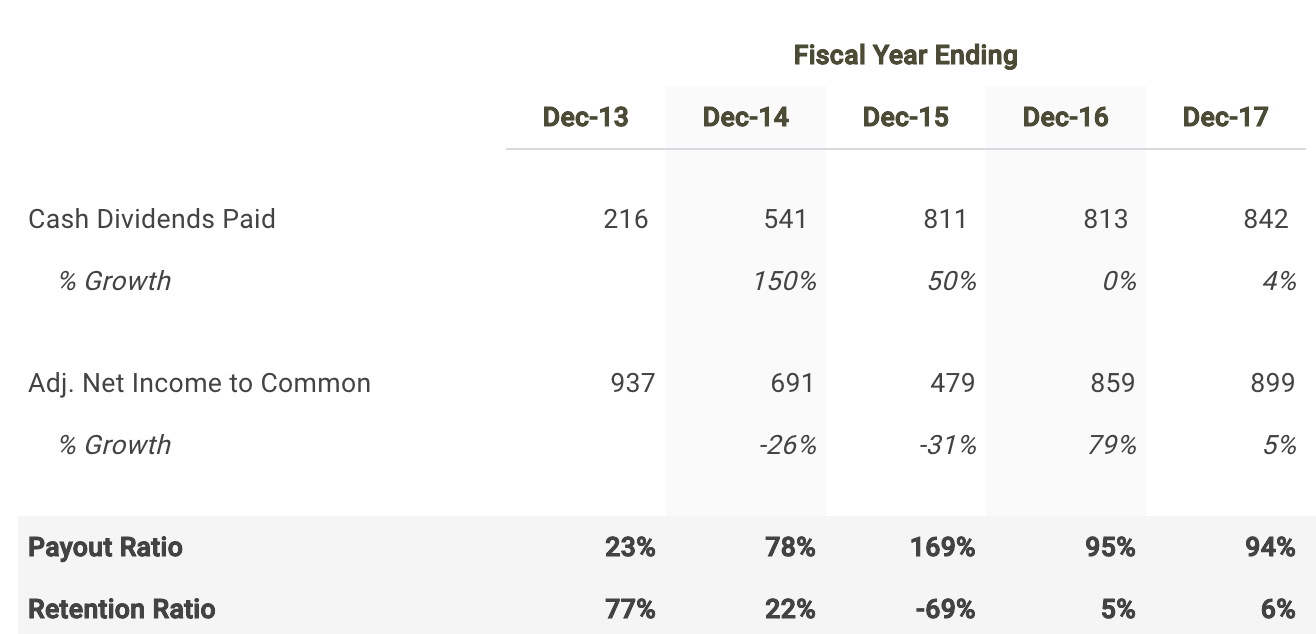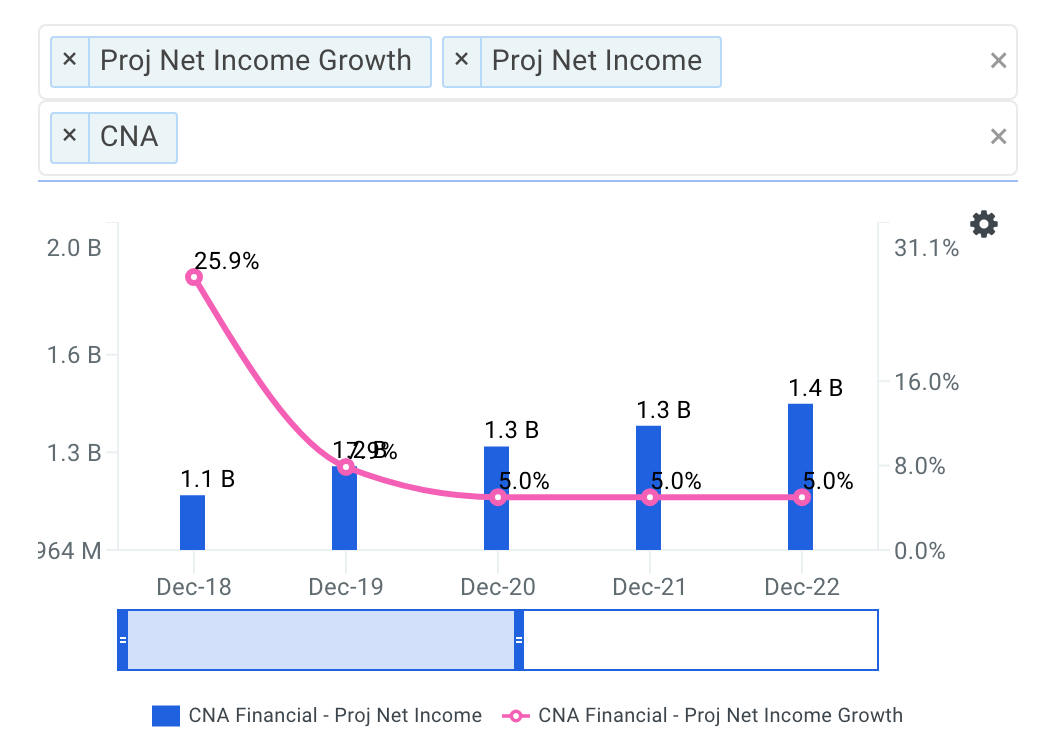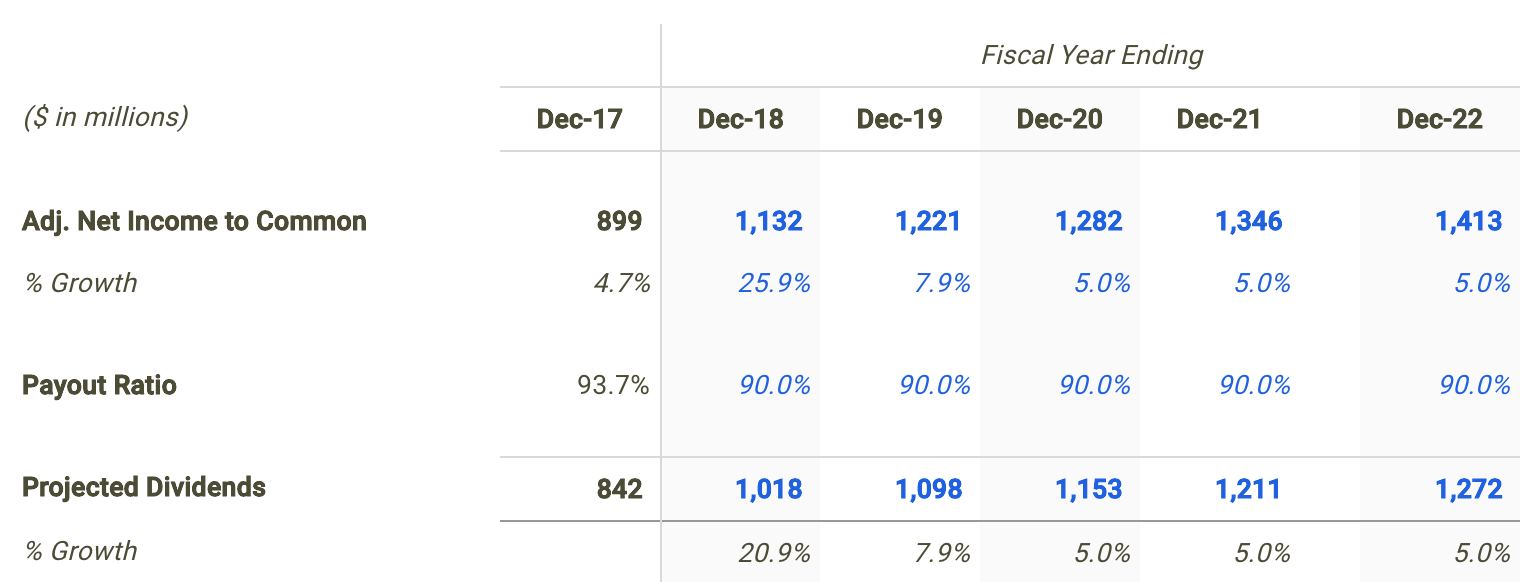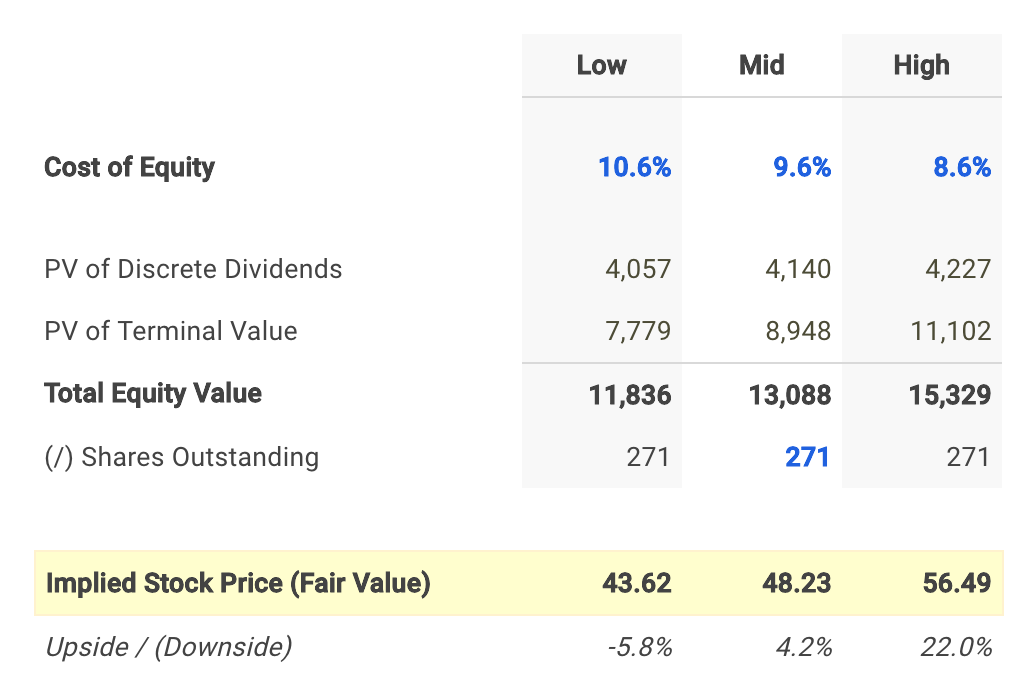Cna Financial Corp (NYSE: CNA) currently pays a dividend yield of 2.6% which is above the Financials sector median of 2.1%. While this makes the total return potential for Cna Financial look attractive, investors may change their mind when analyzing the company's future dividends. In this article, I calculate Cna Financial's fair value by forecasting its dividend distributions and discounting them back to today's value.

##### Valuation Methodologies Are Not Made Equally

The Dividend Discount Model (DDM) estimates the value of a company's stock price based on the theory that its worth is equal to the sum of the present value of its future dividend payments to shareholders.

But how do we know if it's appropriate to use a dividend discount analysis when estimating the fair value of Cna Financial? Many analysts find it difficult when trying to figure out the correct valuation methodology for a given company or are biased towards one specific approach. This is often a mistake which can negatively impact investment decisions and result in trading losses or missed opportunities. No two companies are the same and every business consists of unique characteristics that may require you to adjust your analysis.

Understanding leverage trends is the first step when determining what valuation analyses are relevant for a given company. When a company's leverage doesn’t fluctuate or is expected to remain stable over time, then an equity valuation model (e.g. equity DCF, DDM) will be the most appropriate valuation technique. The reason for this is because when leverage is stable, interest expense on debt can typically be projected with much more reliability.

How do we check if a company's leverage has been fluctuating or is expected to do so? This isn't always straightforward but checking recent debt ratio trends can be a good indicator. Cna Financial's debt to equity ratio has been relatively stable over last few years ranging from 21.8% to 23.7%. This suggests that an equity valuation model is a suitable technique when valuing the company's shares. Now does it make sense to use a dividend discount model knowing that an equity valuation technique is an appropriate methodology?

The next step is to figure out if Cna Financial pays a dividend and if so, is its payout ratio relatively high (typically above 70%)? The table below highlights this information.Source: Cna Financial dividend discount model

Cna Financial distributed a total of \$842 million in cash dividends to shareholders in its most recent fiscal year Dec-17 which represented a payout ratio of 93.7%. It appears that the company meets both criteria. Therefore, it is fitting to use a dividend discount model when determining the fair value of Cna Financial stock.

##### Forecasting Cna Financial's Dividends

The first step in building a dividend discount model is to forecast net income since forecasting dividends directly can be difficult. So let's create a net income forecast for the next five years and use that as the basis for projecting future dividends.

As of June 21, Wall Street analysts are projecting a mediocre growth rate in the company's bottom-line over the next five years. Net income is expected to reach \$1,413 million by fiscal year 2022.Source: Cna Financial Projected Net Income Growth

I use the net income projections above to serve as the basis for my dividend forecast. The next step is to forecast the payout ratio where I selected 90.0% for the next fiscal year which is in line with historical levels.Source: Cna Financial dividend discount model

##### Calculating Cna Financial's Fair Value

The last step is to select a discount rate to calculate the present value of the forecasted dividends. I used finbox.io’s Weighted Average Cost of Capital (WACC) model to help arrive at an estimate for the company's cost of equity.

I determined a reasonable discount rate for Cna Financial to be 9.6% at the midpoint. An updated cost of capital analysis using real-time data can be found at finbox.io's Cna Financial WACC model page.The assumptions used in the dividend discount model calculate a fair value per share for Cna Financial of \$48.23, 4.2% above its current stock price. As a result, investors may conclude that they want to hold off on purchasing shares until the stock develops a wider margin of safety.

##### Conclusion: Dividends Support Stock Price

Discovering the fair value of a company can sometimes be difficult. However, determining an appropriate valuation methodology should not be. Knowing when and when not to use the dividend discount model will help in your investment decision making process.

However, it's important to understand that a dividend discount model will inherently undervalue a stock. This is typically the result of the payout ratio assumption being less than 100% implying some cash leakage. Meaning the approach does not capture value that would otherwise build up as cash on the balance sheet. In practice, this excess retained cash is usually paid out to shareholders as special dividends or to make up for cash shortfalls for future dividends during economic downturns.

Understanding that this approach calculates a conservative fair value estimate may be a promising sign for investors looking to purchase shares or add to an existing position. Since CNA looks to be trading near its intrinsic value based on the analysis above, this could actually represent a 'downside' case meaning an 'upside' case could have a much larger margin of safety.

Note that there are a number of fundamental factors I have not considered in this analysis. I recommend that you continue your research on Cna Financial to gain a better understanding of its future prospects.

As of this writing, I did not hold a position in any of the aforementioned securities and this is not a buy or sell recommendation on any security mentioned.

##### Author: Matt Hogan

Expertise: Valuation, financial statement analysis

Matt Hogan is also a co-founder of finbox.io. His expertise is in investment decision making. Prior to finbox.io, Matt worked for an investment banking group providing fairness opinions in connection to stock acquisitions. He spent much of his time building valuation models to help clients determine an asset’s fair value. He believes that these same valuation models should be used by all investors before buying or selling a stock.

His work is frequently published at InvestorPlace, Benzinga, ValueWalk, AAII, Barron's, Seeking Alpha and investing.com.

Matt can be reached at matt@finbox.io.